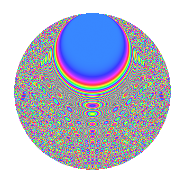# Properties

 Label 3024.2.fzLevel 3024 Weight 2 Character orbit fz Rep. character $$\chi_{3024}(239,\cdot)$$ Character field $$\Q(\zeta_{18})$$ Dimension 648 Sturm bound 1152

# Related objects

## Defining parameters

 Level: $$N$$ = $$3024 = 2^{4} \cdot 3^{3} \cdot 7$$ Weight: $$k$$ = $$2$$ Character orbit: $$[\chi]$$ = 3024.fz (of order $$18$$ and degree $$6$$) Character conductor: $$\operatorname{cond}(\chi)$$ = $$108$$ Character field: $$\Q(\zeta_{18})$$ Sturm bound: $$1152$$

## Dimensions

The following table gives the dimensions of various subspaces of $$M_{2}(3024, [\chi])$$.

Total New Old
Modular forms 3528 648 2880
Cusp forms 3384 648 2736
Eisenstein series 144 0 144

## Trace form

 $$648q + O(q^{10})$$ $$648q - 36q^{33} - 36q^{41} + 36q^{57} + 72q^{65} + 72q^{81} + 108q^{89} + 144q^{93} - 108q^{97} + O(q^{100})$$

## Decomposition of $$S_{2}^{\mathrm{new}}(3024, [\chi])$$ into newform subspaces

The newforms in this space have not yet been added to the LMFDB.

## Decomposition of $$S_{2}^{\mathrm{old}}(3024, [\chi])$$ into lower level spaces

$$S_{2}^{\mathrm{old}}(3024, [\chi]) \cong$$ $$S_{2}^{\mathrm{new}}(108, [\chi])$$$$^{\oplus 6}$$$$\oplus$$$$S_{2}^{\mathrm{new}}(432, [\chi])$$$$^{\oplus 2}$$$$\oplus$$$$S_{2}^{\mathrm{new}}(756, [\chi])$$$$^{\oplus 3}$$

## Hecke Characteristic Polynomials

There are no characteristic polynomials of Hecke operators in the database